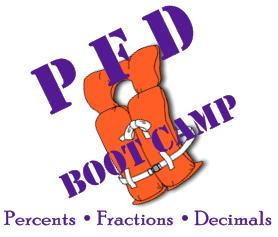About the PFD Boot Camp
Percents, Fractions, and Decimals

# Overview

The PFD Boot Camp is a series of materials to help students with Percents, Fractions, and Decimals. PFD Boot Camp has:

• Worked Examples - Problems are solved step-by-step.
• Videos - Instructional (YouTube) videos explaining concepts, procedures, and problem solving. (Videos can be found also inside the Modules.)
• Modules - Complete instruction on a topic. Includes worked examples, videos, and self-checks. Each module covers from 1 to 5 objectives.

The purpose is to help students develop understanding, competence, and confidence with percents, fractions, and decimals.

Worked Examples, Videos, and Full Modules can be accessed using the Index Page.

This site is under construction.

• Module #1 is complete
• Module #2 is complete
• Module #3 is complete
• Module #4 is complete## Goals

The goals are to help students:

• Understand the meanings of, and relationships between, fractions, decimals, and percents,
• Understand the uses of fractions, decimals, and percents in the real world,
• Develop computational skills and confidence working with fractions, decimals, and percents.
• Apply computational skills to fluently solve real-world problems.

## Intended Audiences

These modules are intended for students who "have had" fractions, decimals, and percents, but want to brush up and improve their understanding and skills. Intended audiences include:

• Teacher education students,
• College students,
• Junior or senior high students,
• Anyone who wants to improve their understanding and skills with fractions, decimals, and percents.

## In Each Module

In each of the modules you will find

• A list of the objectives covered in the module, and why these are important concepts and skills,
• A (short) 6-question pre-quiz, which can be used to see how well you understand the material at the beginning,
• An explanation of how to do the computations and why it works,
• Exercises to check your understanding,
• Practice items,
• A (short) 6-question post-quiz, which can be used to see how well you understand the material after working through the module.

## List of Modules

This project is under construction. The intention is to develop nine (9) modules.

The first four modules are:

1. Fractions – what are they?

a. Meaning of a fraction.

b. Put fraction in lowest terms.

c. Put fraction in higher terms.

d. Fraction to division relationship (¾ = 3÷4).

e. Mixed number to improper fraction.

2. Decimals – what's that dot for?

a. Meaning of decimals.

b. Read a decimal correctly.

c. Add and subtract decimals.

d. Convert decimals to fractions.

e. Multiply and divide numbers (& decimals) by 10, 100, 1000, ....

3. Multiply Decimals – hey, count from the right, not the left

a. Multiply decimals.

4. Long Division – Daddy, Mommy, Sister, Brother

a. "Long" Division Algorithm.

b. Improper fraction to mixed number.

c. "Long" division algorithm with decimals.

d. Convert fractions (& mixed) to decimals.

## In-person Help Sessions

PFD Boot Camp Help Sessions - to be announced.

## Need Practice or Help with the Basic Facts?

Click here for Basic Training (on the basic facts of addition, subtraction, multiplication, and division).